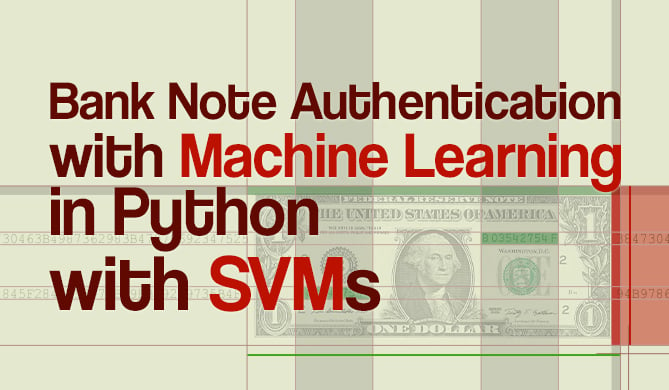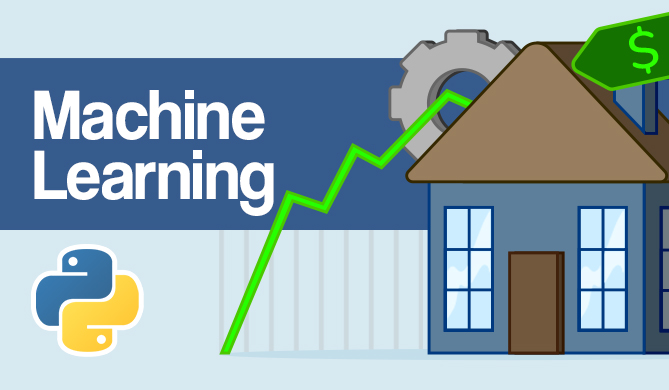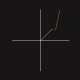K-Means Elbow Method and Silhouette Analysis with Yellowbrick and Scikit Learn

# K-Means Elbow Method and Silhouette Analysis with Yellowbrick and Scikit Learn## Introduction

K-Means is one of the most popular clustering algorithms. By having central points to a cluster, it groups other points based on their distance to that central point.

A downside of K-Means is having to choose the number of clusters, K, prior to running the algorithm that groups points.

If you'd like to read an in-depth guide to K-Means Clustering, take a look at "K-Means Clustering with Scikit Learn".

## Elbow Method and Silhouette Analysis

The most commonly used techniques for choosing the number of Ks are the Elbow Method and the Silhouette Analysis.

To facilitate the choice of Ks, the Yellowbrick library wraps up the code with for loops and a plot we would usually write into 4 lines of code.

To install Yellowbrick directly from a Jupyter notebook, run:

! pip install yellowbrick


Let's see how it works for a familiar dataset which is already part of Scikit Learn, the Iris dataset.

The first step is to import the dataset, KMeans and yellowbrick libraries, and load the data:

from sklearn.datasets import load_iris
from sklearn.cluster import KMeans
from yellowbrick.cluster import KElbowVisualizer, SilhouetteVisualizer



Notice here, we import the KElbowVisualizer and SilhouetteVisualizer from yellowbrick.cluster, those are the modules we'll use to visualize Elbow and Silhouette results!

After loading the dataset, in the data key of the bunch (a data type which is an extension of a dictionary) are the values of the points we want to cluster. If you want to know what the numbers represent, take a look at iris['feature_names'].

It is known that the Iris dataset contains three types of irises: 'versicolor', 'virginica' and 'setosa'. You can also inspect the classes in iris['target_names'] to verify.

So, we have 4 features to cluster and they should be separated into 3 different clusters according to what we already know. Let's see if our results with the Elbow Method and Silhouette Analysis will corroborate that.

First, we will select the feature values:

print(iris['feature_names']) # displays ['sepal length (cm)', 'sepal width (cm)', 'petal length (cm)', 'petal width (cm)']
print(iris['target_names']) # displays array(['setosa', 'versicolor', 'virginica'], dtype='<U10')

X = iris['data']


Then, we can create a KMeans model, a KElbowVisualizer() instance which will receive that model along with the number of ks for which a metric will be computed, in this case from 2 to 11 Ks.

After that, we fit the visualizer with the data using fit() and display the plot with show(). If a metric is not specified, the visualizer uses the distortion metric, which computes the sum of squared distances from each point to its assigned center:

model = KMeans(random_state=42)

elb_visualizer = KElbowVisualizer(model, k=(2,11))
elb_visualizer.fit(X)
elb_visualizer.show()


Now, we already have a Distortion Score Elbow for KMeans Clustering plot with a vertical line marking which would be the best number of ks, in this case, 4.

Seems the Elbow Method with a distortion metric wasn't the best choice if we didn't know the actual number of clusters. Will Silhouette also indicate that there are 4 clusters? To answer that, we just need to repeat the last code with a model with 4 clusters and a different visualizer object:

model_4clust = KMeans(n_clusters = 4, random_state=42)

sil_visualizer = SilhouetteVisualizer(model_4clust)
sil_visualizer.fit(X)
sil_visualizer.show()


The code displays a Silhouette Plot of KMeans Clustering for 150 Samples in 4 Centers. To analyze these clusters, we need to look at the value of the silhouette coefficient (or score), its best value is closer to 1. The average value we have is 0.5, marked by the vertical line, and not so good.

We also need to look at the distribution between clusters - a good plot has similar sizes of clustered areas or well-distributed points. In this graph, there are 3 smaller clusters (number 3, 2, 1) and one larger cluster (number 0), which isn't the result we were expecting.

Free eBook: Git Essentials

Check out our hands-on, practical guide to learning Git, with best-practices, industry-accepted standards, and included cheat sheet. Stop Googling Git commands and actually learn it!

Let's repeat the same plot for 3 clusters to see what happens:

model_3clust = KMeans(n_clusters = 3, random_state=42)

sil_visualizer = SilhouetteVisualizer(model_3clust)
sil_visualizer.fit(X)
sil_visualizer.show()


By changing the number of clusters, the silhouette score got 0.05 higher and the clusters are more balanced. If we didn't know the actual number of clusters, by experimenting and combining both techniques, we would have chosen 3 instead of 2 as the number of Ks.

This is an example of how combining and comparing different metrics, visualizing data, and experimenting with different values of clusters is important to lead the result in the right direction. And also, how having a library that facilitates that analysis can help in that process!

Last Updated: November 8th, 2023

Get tutorials, guides, and dev jobs in your inbox.

Cássia SampaioAuthor

Data Scientist, Research Software Engineer, and teacher. Cassia is passionate about transformative processes in data, technology and life. She is graduated in Philosophy and Information Systems, with a Strictu Sensu Master's Degree in the field of Foundations Of Mathematics.Project

### Bank Note Fraud Detection with SVMs in Python with Scikit-Learn

# python# machine learning# scikit-learn# data science

Can you tell the difference between a real and a fraud bank note? Probably! Can you do it for 1000 bank notes? Probably! But it...DetailsProject

### Hands-On House Price Prediction - Machine Learning in Python

# python# machine learning# scikit-learn# tensorflow

If you've gone through the experience of moving to a new house or apartment - you probably remember the stressful experience of choosing a property,...Details